# mne.SourceMorph#

class mne.SourceMorph(subject_from, subject_to, kind, zooms, niter_affine, niter_sdr, spacing, smooth, xhemi, morph_mat, vertices_to, shape, affine, pre_affine, sdr_morph, src_data, vol_morph_mat, *, verbose=None)[source]#

Morph source space data from one subject to another.

Note

This class should not be instantiated directly. Use `mne.compute_source_morph()` instead.

Parameters:
subject_from

Name of the subject from which to morph as named in the SUBJECTS_DIR.

subject_to

Name of the subject on which to morph as named in the SUBJECTS_DIR. The default is ‘fsaverage’. If morphing a volume source space, subject_to can be the path to a MRI volume. Can also be a list of two arrays if morphing to hemisphere surfaces.

kind

Kind of source estimate. E.g. ‘volume’ or ‘surface’.

zooms
niter_affine

Number of levels (`len(niter_affine)`) and number of iterations per level - for each successive stage of iterative refinement - to perform the affine transform.

niter_sdr

Number of levels (`len(niter_sdr)`) and number of iterations per level - for each successive stage of iterative refinement - to perform the Symmetric Diffeomorphic Registration (sdr) transform .

spacing
smooth
xhemibool

Morph across hemisphere.

morph_mat`scipy.sparse.csr_matrix`

The sparse surface morphing matrix for spherical surface based morphing .

vertices_to

The destination surface vertices.

shape`tuple`

The volume MRI shape.

affine`ndarray`

The volume MRI affine.

pre_affineinstance of `dipy.align.AffineMap`

The transformation that is applied before the before `sdr_morph`.

sdr_morphinstance of `dipy.align.DiffeomorphicMap`

The class that applies the the symmetric diffeomorphic registration (SDR) morph.

src_data`dict`

Additional source data necessary to perform morphing.

vol_morph_mat

The volumetric morph matrix, if `compute_vol_morph_mat()` was used.

verbose

Control verbosity of the logging output. If `None`, use the default verbosity level. See the logging documentation and `mne.verbose()` for details. Should only be passed as a keyword argument.

Notes

New in version 0.17.

References

Methods

 `apply`(stc_from[, output, mri_resolution, ...]) Morph source space data. `compute_vol_morph_mat`(*[, verbose]) Compute the sparse matrix representation of the volumetric morph. `save`(fname[, overwrite, verbose]) Save the morph for source estimates to a file.
apply(stc_from, output='stc', mri_resolution=False, mri_space=None, verbose=None)[source]#

Morph source space data.

Parameters:
stc_from

The source estimate to morph.

output`str`

Can be ‘stc’ (default) or possibly ‘nifti1’, or ‘nifti2’ when working with a volume source space defined on a regular grid.

mri_resolution

If True the image is saved in MRI resolution. Default False. WARNING: if you have many time points the file produced can be huge. The default is mri_resolution=False.

mri_space

Whether the image to world registration should be in mri space. The default (None) is mri_space=mri_resolution.

verbose

Control verbosity of the logging output. If `None`, use the default verbosity level. See the logging documentation and `mne.verbose()` for details. Should only be passed as a keyword argument.

Returns:
stc_to

The morphed source estimates.

Examples using `apply`: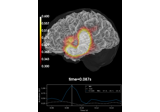Source reconstruction using an LCMV beamformer

Source reconstruction using an LCMV beamformer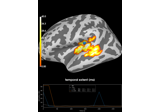2 samples permutation test on source data with spatio-temporal clustering

2 samples permutation test on source data with spatio-temporal clustering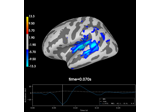Cross-hemisphere comparison

Cross-hemisphere comparison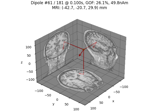Compute sparse inverse solution with mixed norm: MxNE and irMxNE

Compute sparse inverse solution with mixed norm: MxNE and irMxNE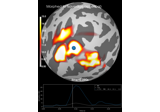Morph surface source estimate

Morph surface source estimate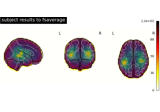Morph volumetric source estimate

Morph volumetric source estimate
compute_vol_morph_mat(*, verbose=None)[source]#

Compute the sparse matrix representation of the volumetric morph.

Parameters:
verbose

Control verbosity of the logging output. If `None`, use the default verbosity level. See the logging documentation and `mne.verbose()` for details. Should only be passed as a keyword argument.

Returns:
morphinstance of `SourceMorph`

The instance (modified in-place).

Notes

For a volumetric morph, this will compute the morph for an identity source volume, i.e., with one source vertex active at a time, and store the result as a `sparse` morphing matrix. This takes a long time (minutes) to compute initially, but drastically speeds up `apply()` for STCs, so it can be beneficial when many time points or many morphs (i.e., greater than the number of volumetric `src_from` vertices) will be performed.

When calling `save()`, this sparse morphing matrix is saved with the instance, so this only needs to be called once. This function does nothing if the morph matrix has already been computed, or if there is no volume morphing necessary.

New in version 0.22.

Examples using `compute_vol_morph_mat`:Morph volumetric source estimate

Morph volumetric source estimate
save(fname, overwrite=False, verbose=None)[source]#

Save the morph for source estimates to a file.

Parameters:
fname`str`

The stem of the file name. ‘-morph.h5’ will be added if fname does not end with ‘.h5’.

overwritebool

If True (default False), overwrite the destination file if it exists.

verbose

Control verbosity of the logging output. If `None`, use the default verbosity level. See the logging documentation and `mne.verbose()` for details. Should only be passed as a keyword argument.

## Examples using `mne.SourceMorph`#Source reconstruction using an LCMV beamformer

Source reconstruction using an LCMV beamformer2 samples permutation test on source data with spatio-temporal clustering

2 samples permutation test on source data with spatio-temporal clusteringCross-hemisphere comparison

Cross-hemisphere comparisonCompute sparse inverse solution with mixed norm: MxNE and irMxNE

Compute sparse inverse solution with mixed norm: MxNE and irMxNEMorph surface source estimate

Morph surface source estimateMorph volumetric source estimate

Morph volumetric source estimate